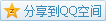# 函数

## 阐明 Exposition

• 函数 (function)

• 有集合$X,Y$，一个从$X$$Y$函数即是一个关系$f$满足$\mathrm{dom}f=X$并且对于每一个$x\in X$都存在唯一的$y\in Y$使$(x,y)\in f$.
• $y$唯一性

• $y\in Y$唯一性的表述: $(x,y)\in f\land (x,z)\in f \Rightarrow y=z$.
• 满足上述唯一性的$y\in Y$采用$f(x)$记号表示.
• 这样的关系以$y=f(x)$写法取代$x\ f\ y$$(x,y)\in f$的一般性写法.
• 自变量

• 某一个元素$y\in Y$被称作是函数$f$自变量$x$时的.
• 也称$f$$x$变换映射$y$.
• 潜在的同义词

• 映射 (map, mapping)
• 变换 (transformation)
• 对应 (correspondence)
• 算子 (operator)
• 函数的记号

• $f: X\rightarrow Y$便表明$f$是一个从$X$$Y$的函数.
• 函数的集合

• 所有从$X$$Y$的函数组成的集合，是$\mathcal{P}(X\times Y)$的子集，记作$Y^X$.
• 如果$A$$X$的子集，则$\forall y\in Y, \exists x\in A, f(x)=y$构成的$Y$的子集被称作是$A$$f$下的像，记作$f(A)$.
• 定义域值域

• 根据笛卡儿积的投影，一个从$X$$Y$的函数的定义域$X$，但它的值域$f(X)$的未必是$Y$.
• 陪域满射

• 一个从$X$$Y$的函数，$Y$叫做陪域，如果函数$f$的值域和陪域相同，则称函数$f$满射.
• 包含映射 (inclusion map)

• 如果$X\subset Y$，那么从$X$$Y$的函数$f(x)=x, x\in X$就是一个从$X$$Y$包含映射.
• 恒等映射 (identity map)

• $X$$X$包含映射被称为$X$上的恒等映射.
• 限制扩充

• 已知$f: Y\rightarrow Z, X\subset Y$$g(x)=f(x), x\in X$，则$g$被称为$f$$X$限制$f$被称为$g$$Y$扩充.
• 写作$(f|X)(x)=f(x), x\in X$，同时$(f|X)=f(X)$也成立.
• $Y$的子集$X$的包含映射即是在$Y$上的恒等映射到$X$的限制.
• 投影 (projection)

• 如果$f: X\times Y\rightarrow X$$f(x,y)=x$，则$f$被称作是从$X\times Y$$X$上的投影.
• 正则映射自然映射） (canonical map)

• 如果$f: X\rightarrow X/R$$f(x)=x/R$，那么$f$被称为从$X$$X/R$正则映射，也叫自然映射**.
• 双射一一对应） (one-to-one correspondence)

• 考虑一个从$X$满射$Y$的函数$f$，定义$X$上的等价关系$R$，有$a,b\in X\land f(a) = f(b), a\ R\ b$. 对于每一个$y\in Y$，使得$g(y)$是一个由所有$x\in X, f(x)=y$构成的集合. 由$R$的定义可知，对于每一个$y$$g(y)$必然是$R$的一个等价类.
• 比较有趣的是$g(x)$的性质，如果$u,v\in Y, u\neq v$那么$g(u),g(v)\in X/R, g(u)\neq g(v)$. 这样一种将不同的元素映射至不同元素的函数称为双射.
• 自然数（初步）

• 定义$0=\varnothing, 1=\{\varnothing\}, 2=\{\varnothing, \{\varnothing\}\}$.
• 也就是说$0=\varnothing, 1=\{0\}, 2=\{0,1\}$.
• 发现$card(0), card(1), card(2)$即元素个数分别代表各自的数目.
• 指示函数（避免与概率论的特征函数歧义）(characteristic function)
• 已知$A\subset X$，则$A$指示函数$\chi_A(x)$可以如下定义$$\chi_A(x)=\begin{cases}1, &x\in A\\0, &x\in X-A\end{cases}$$
• 指示函数$\mathcal{P}(X)$$2^X$之间的一一对应函数.

## 习题 Exercise

• 证明无论$Y$是否为空，$Y^{\varnothing}$只有一个元素$\varnothing$.
\begin{aligned}
\mathbf{i.} \ &f=\varnothing\\
\Rightarrow &\mathrm{dom}f=\varnothing\land (\forall x,\exists y\in Y: (x,y)\in f)\\
\Rightarrow &f:\varnothing\rightarrow Y\\
\Rightarrow & \varnothing\in Y^\varnothing\\
\therefore& \{\varnothing\}\subset Y^\varnothing\\
\mathbf{ii.} \ &Y^\varnothing\subset \mathcal{P}(\varnothing\times Y) = \{\varnothing\}\\
\therefore &Y^\varnothing \subset \{\varnothing\}\\
&\mathrm{Q.E.D.}
\end{aligned}
• 证明$X$非空时，${\varnothing}^X$为空.
\begin{aligned}
& f\in \varnothing^X\\
\Rightarrow &\forall a\in X,\exists b:(a,b)\in f, X\neq \varnothing\\
\Rightarrow &\varnothing^X=\varnothing\\
&\mathrm{Q.E.D.}
\end{aligned}

思考: 其实在习题里已经能够看到自然数幂的身影了.